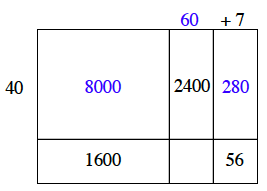### Home > CC1MN > Chapter 2 Unit 2 > Lesson CC1: 2.3.3 > Problem2-75

2-75.

Solve each generic-rectangle puzzle. For each part, write a multiplication sentence, as you did in problem 2-72.

1.  $+4$ $30$ $1500$ $250$
• What do you need to multiply $30$ by to get $1500$? This will give you the top edge length of the rectangle. Can you then figure out what must go below the 30 in order to get the area of 250?

1.  $+7$ $40$ $2400$ $1600$ $56$
• Some missing parts have been filled in. Do they make sense? Now try to fill in more parts yourself.